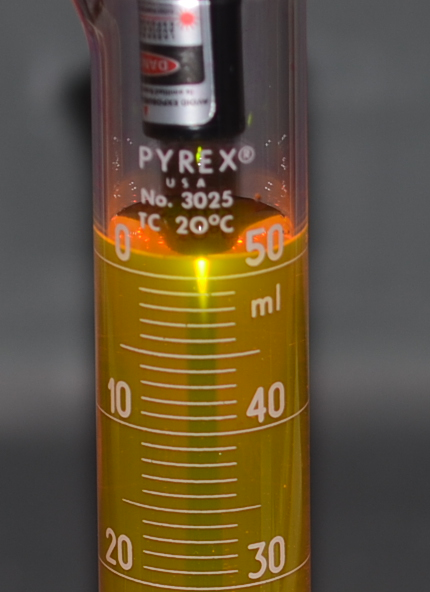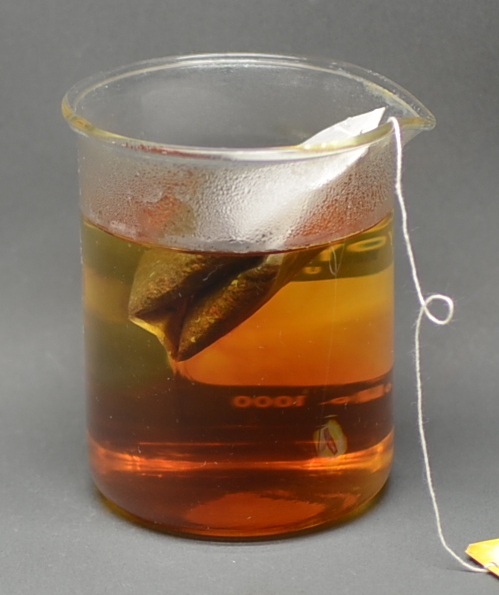Illustrated Glossary of Organic Chemistry

Beer's Law (Beer-Lambert Law): The amount of energy absorbed or transmitted by a solution is proportional to the solution's molar absorptivity and the concentration of solute. In simple terms, a more concentrated solution absorbs more light than a more dilute solution does. Mathematical statement of Beer's law is A = εlc, where: A = absorption; ε = molar attenuation coefficient, l = path length (the thickness of the solution), and c = concentration of the solution.A demonstration of Beer's Law. A aqueous solution of rhodamine (a fluorescent dye) is illuminated with a green laser pointer. Note how far the green light travels before it is fully absorbed (the length of the yellow-green line).The same green laser illuminating an aqueous rhodamine solution of lower concentration. Note the laser light is visible to a greater depth than observed in the photo above, because the less concentrated solution absorbs the light less efficiently (i.e., the more dilute solution has lower c).Time = 0 minutes Time = 1 minute Time = 2 minutes Time = 3 minutes Time = 4 minutes
Beer's law in action. While brewing tea, the color darkens as time progresses because the concentration of tannin (the brown material) increases as it dissolves out of the leaves in the tea bag. Click here to see a time lapse movie.